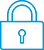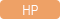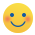cancel
Showing results for
Did you mean:It has been a while since anyone has replied. Simply ask a new question if you would like to start the discussion again.New member
2 1 0 0
Message 1 of 4
1,160
Flag Post

Solved!

# Defect in HP10s+ calculatorHP Recommended
HP10s+

Hi, I'd like to report a defect in the HP10s+ calculator. When performing the following sentence:

(5+2*sq.root5)^2-10*(5+2sq.root5)+5 =

The answer should be 0 (zero), but the calculator shows 3.76*10^-12

That's weird.

Tags (1)
1 ACCEPTED SOLUTION

Accepted SolutionsLevel 8
747 744 121 188
Message 2 of 4
Flag PostHP Recommended

Thanks for the post!Yes it is weird, but not necessarily a defect! This is a very great "why is it doing this?" question actually.

This type of behavior is inherent in any system that uses finite precision numerical values. We as humans can look at something an easily determine "that cancels out", but when you numerically evaluate things there will ALWAYS be slight errors in the end of intermediate numbers. That error can be propogated through in continuing calculations and doesn't necessarily cancel out in the end.

Every single calculator (or any math software) ever made will exhibit similar things - not always for the same types of input, but it is always there if you know how to expose it through carefully crafted calculations. There are often all sorts of tricks or techniques in order to minimize or hide these imprecisions built into the software.

In fact, mathematicians have PROVEN (as in a^2+b^2=c^2 type of PROVEN) that it is **impossible** to create a computer algorithm that will perfectly plot a function in all cases or inputs.

While I agree with you that it should be 0 in a "perfect" calculation, the fact that the result is "almost 0" does not necessarily mean there is a bug or problem here.

Here's a great page (http://www.rskey.org/~mwsebastian/miscprj/results.htm) that does a comparison of evaluating arcsin (arccos (arctan (tan (cos (sin (9) ) ) ) ) )  for a bunch of different calculators. It is quite interesting to see the wide differences in the results.

Why the Forensics Algorithm Produces Different Results on Different Calculators

Calculators use approximations in computing transcendental functions. Most calculators use the CORDIC (COordinate Rotation DIgital Computer) method to approximate their transcendental functions. A few calculators use polynomial approximations. Whichever method is used, the accuracy of the approximation is going to generally be limited by the precision of the calculator (the number of digits the calculator is capable of calculating) and the quality of the software written to implement the algorithm performing the approximation.

Against this backdrop, we can better understand why the forensics algorithm produces such a variety of results on different calculators. When evaluating the forensics algorithm, several factors combine to produce the observed inaccuracies. These factors include:

• Inherent Loss of Precision
• Number of Digits Calculated (Precision)
• Algorithm Quality (Accuracy)

The first factor is inherent to the forensics algorithm and independent of which calculator is being evaluated. The latter two factors, which are dependent on the calculator being evaluated, are the variables responsible for the varying results produced by the forensics algorithm.

TW

Although I work for the HP calculator group as a head developer of the HP Prime, the views and opinions I post here are my own.
Tags (1)
3 REPLIES 3Level 8
747 744 121 188
Message 2 of 4
Flag PostHP Recommended

Thanks for the post!Yes it is weird, but not necessarily a defect! This is a very great "why is it doing this?" question actually.

This type of behavior is inherent in any system that uses finite precision numerical values. We as humans can look at something an easily determine "that cancels out", but when you numerically evaluate things there will ALWAYS be slight errors in the end of intermediate numbers. That error can be propogated through in continuing calculations and doesn't necessarily cancel out in the end.

Every single calculator (or any math software) ever made will exhibit similar things - not always for the same types of input, but it is always there if you know how to expose it through carefully crafted calculations. There are often all sorts of tricks or techniques in order to minimize or hide these imprecisions built into the software.

In fact, mathematicians have PROVEN (as in a^2+b^2=c^2 type of PROVEN) that it is **impossible** to create a computer algorithm that will perfectly plot a function in all cases or inputs.

While I agree with you that it should be 0 in a "perfect" calculation, the fact that the result is "almost 0" does not necessarily mean there is a bug or problem here.

Here's a great page (http://www.rskey.org/~mwsebastian/miscprj/results.htm) that does a comparison of evaluating arcsin (arccos (arctan (tan (cos (sin (9) ) ) ) ) )  for a bunch of different calculators. It is quite interesting to see the wide differences in the results.

Why the Forensics Algorithm Produces Different Results on Different Calculators

Calculators use approximations in computing transcendental functions. Most calculators use the CORDIC (COordinate Rotation DIgital Computer) method to approximate their transcendental functions. A few calculators use polynomial approximations. Whichever method is used, the accuracy of the approximation is going to generally be limited by the precision of the calculator (the number of digits the calculator is capable of calculating) and the quality of the software written to implement the algorithm performing the approximation.

Against this backdrop, we can better understand why the forensics algorithm produces such a variety of results on different calculators. When evaluating the forensics algorithm, several factors combine to produce the observed inaccuracies. These factors include:

• Inherent Loss of Precision
• Number of Digits Calculated (Precision)
• Algorithm Quality (Accuracy)

The first factor is inherent to the forensics algorithm and independent of which calculator is being evaluated. The latter two factors, which are dependent on the calculator being evaluated, are the variables responsible for the varying results produced by the forensics algorithm.

TW

Although I work for the HP calculator group as a head developer of the HP Prime, the views and opinions I post here are my own.
Tags (1)New member
2 1 0 0
Message 3 of 4
Flag PostHP Recommended

Thanks for the explanation. I didn't know about that. I thought it was a bug because when trying on other HP calculators it would give me the right answer (0). Good to know!Level 8
650 635 124 222
Message 4 of 4
Flag PostHP Recommended

Fun factoid: Only the modern HP calculators (which have 12-digit mantissas) get a result of 0. If you try that calculation on HP's older calculators (e.g. HP-41) which have 10-digit mantissas, the result is -1E-08.  This is just an example of what Tim explained above; it's not a bug but rather an inevitable result of finite-precision rounding and error accumulation.

-Joe-
† The opinions expressed above are the personal opinions of the authors, not of HP. By using this site, you accept the Terms of Use and Rules of Participation